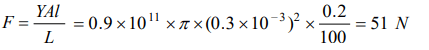## Mechanical Properties of Solids Questions and Answers Part-9

1. An iron rod of length 2m and cross section area of $50mm^{2}$ , stretched by 0.5 mm, when a mass of 250 kg is hung from its lower end. Young's modulus of the iron rod is
a) $19.6 \times 10^{10}N\diagup m^{2}$
b) $19.6 \times 10^{15}N\diagup m^{2}$
c) $19.6 \times 10^{18}N\diagup m^{2}$
d) $19.6 \times 10^{20}N\diagup m^{2}$

Explanation: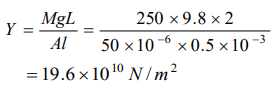2. In solids, inter-atomic forces are
a) Totally repulsive
b) Totally attractive
c) Combination of (a) and (b)
d) None of these

Explanation: Combination of (a) and (b)

3. A force F is applied on the wire of radius r and length L and change in the length of wire is l. If the same force F is applied on the wire of the same material and radius 2r and length 2L, Then the change in length of the other wire is
a) l
b) 2l
c) l/2
d) 4l

Explanation: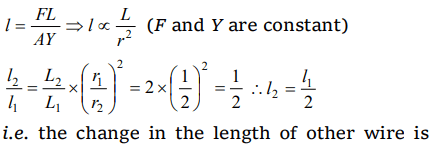$\frac{l}{2}$

4. The modulus of elasticity is dimensionally equivalent to
a) Surface
b) Stress
c) Strain
d) None of these

Explanation: Stress

5. Under elastic limit the stress is
a) Inversely proportional to strain
b) Directly proportional to strain
c) Square root of strain
d) Independent of strain

Explanation: Directly proportional to strain

a) 4 mm
b) 16 mm
c) 1 mm
d) 0.25 mm

Explanation: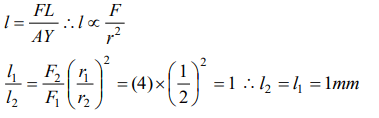7. The units of Young ‘s modulus of elasticity are
a) $N m^{-1}$
b) N-m
c) $N m^{-2}$
d) $N m^{2}$

Explanation: $N m^{-2}$

8.Two similar wires under the same load yield elongation of 0.1 mm and 0.05 mm respectively. If the area of cross- section of the first wire is $4mm^{2}$ then the area of cross section of the second wire is
a) $6mm^{2}$
b) $8mm^{2}$
c) $10mm^{2}$
d) $12mm^{2}$

Explanation: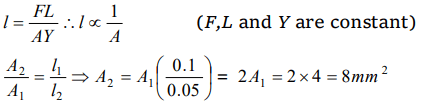9. A 5 m long aluminium wire $\left(Y=7 \times 10^{10}N\diagup m^{2}\right)$    of diameter 3 mm supports a 40 kg mass. In order to have the same elongation in a copper wire $\left(Y=12 \times 10^{10}N\diagup m^{2}\right)$    of the same length under the same weight, the diameter should now be, in mm.
a) 1.75
b) 1.5
c) 2.5
d) 5.0